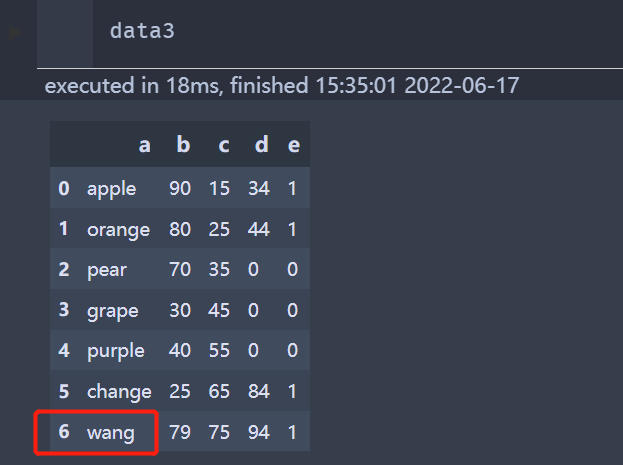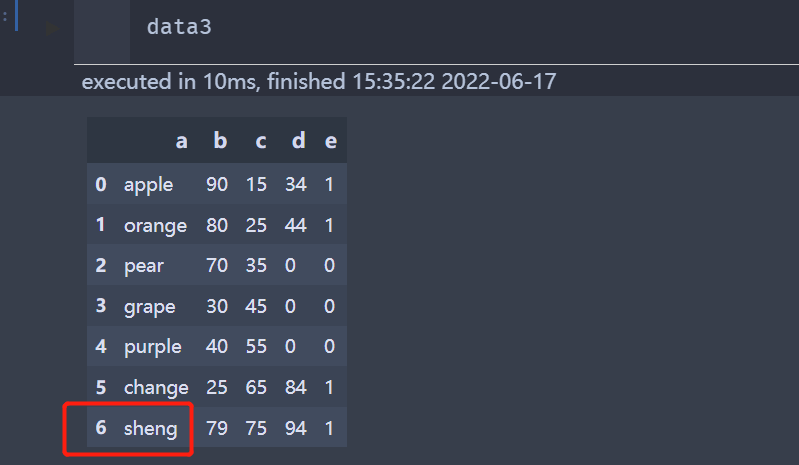# pandas Dataframe實現批量修改值的方法

## 1.使用iloc對數據進行批量修改```import pandas as pd

data1 = data
data1.iloc[2:5,3:] = 0
data1```.iloc用法[],先行後列,並且都是不包含最後一個元素，例如取[2,3,4]就是[2:5],列同樣遵循此規則

## 2.對數據進行判定後,相互+/-/某個數*

### 第一種方法：使用內置函數where函數

`Series.where(cond, other=nan, inplace=False, axis=None, level=None, errors='rais',...)`

```# data2為data數據的一部分
data2 = data.iloc[0:,1:]
print(data2)
data2.where(data2>25, data2+5,inplace=True)````mask(cond, other=nan)`
• where：替換條件(condition)為False處的值

`data2.mask(data2<25, data2+5, inplace=True)`### 第三種方法：replace函數

replace可以替換文本值，也可以使用字典替換多個值,也可以使用正則表達式嵌套方法,替換很多不同的值

```# 替換文本值
data3 = data
data3.replace('wange', 'sheng', inplace=True)
data3``````# 替換多個值
# 將所有的0和1互換
data3.replace({1:0,0:1},inplace=True)``````# 切記使用正則表達式的時候,一定要添加上regex=True
data3.replace('[a-zA-Z]+','Anonymous',regex=True,inplace=True)```